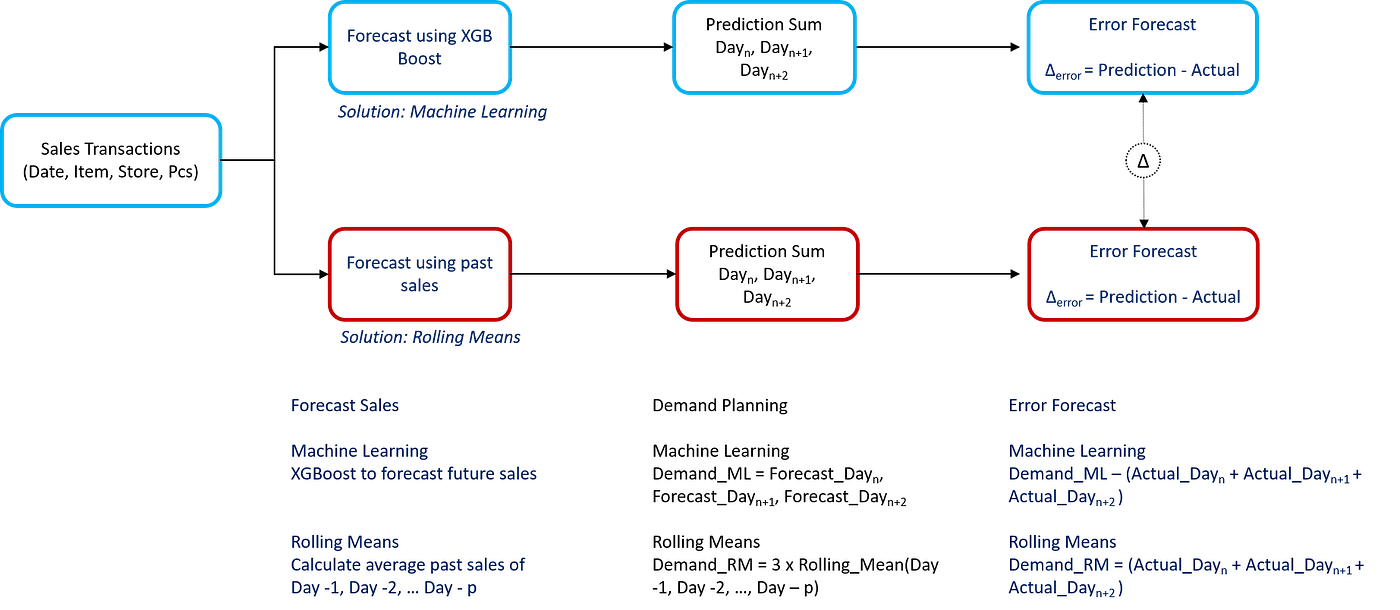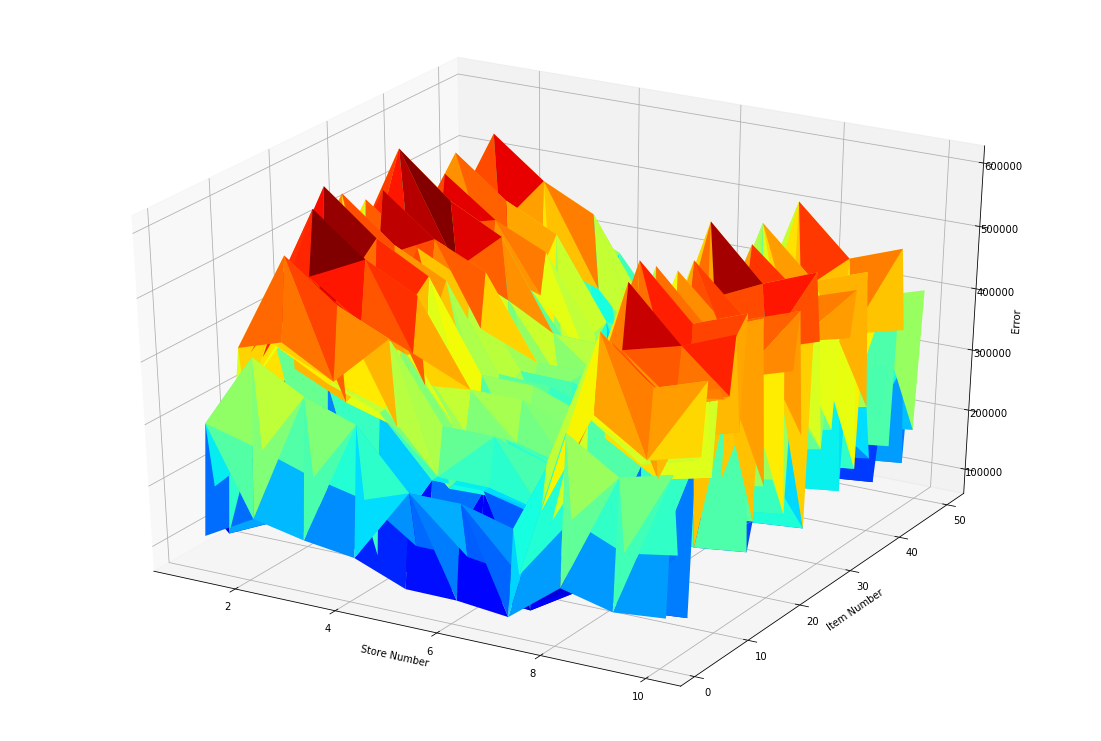# Machine Learning for Retail Demand Forecasting

Comparative study of Demand Forecasting Methods for a Retail Store (XGBoost Model vs. Rolling Mean)

Comparative study of Demand Forecasting Methods for a Retail Store (XGBoost Model vs. Rolling Mean)

Article originally published on Medium.

### I. Demand Planning Optimization Problem Statement

For most retailers, demand planning systems take a fixed, rule-based approach to forecast and replenishment order management.

Such an approach works well enough for stable and predictable product categories but can show its limits regarding Inventory and Replenishment Optimization.

This potential optimization can reduce operational costs by:

• Inventory Optimization: matching store inventory with actual needs to reduce storage space needed (Rental Costs)
• Replenishment Optimization: optimizing replenishment quantity per order to minimize the number of replenishments between warehouse and stores (Warehousing & Transportation Costs)

Example: Retailer with 50 Stores

For this study, we’ll take a dataset from the Kaggle challenge: Store Item Demand Forecasting Challenge.

Scope

• Transactions from 2013–01–01 to 2017–12–31
• 913,000 Sales Transactions
• 50 unique SKU
• 10 Stores

### (Update) Improve the model

I have been working on an improved version of the model and I share my insights in another article with the full code shared in this repository.

🔗

The goal is to understand the impact of adding business features (price change, sales trend, store closing, …) on the accuracy of the model.

## II. XGBoost for Sales Forecasting

The initial dataset has been used for a Kaggle Challenge where teams were competing to design the best model to predict sales.

The first objective here is to design a prediction model using XGBoost; this model will be used to optimize our replenishment strategy ensuring inventory optimization and reducing the number of deliveries from your Warehouse.

### 4. Heat Map to check correlation

Let us keep the monthly average since it has the highest correlation with sales; and remove other features highly correlated to each other.

### 6. Results Prediction Model

Based on this prediction model, we’ll build a simulation model to improve demand planning for store replenishment.

DataFrame Features

• date: Transaction date
• item: SKU Number
• store: Store Number
• sales: Actual value of sales transaction
• sales_prd: XGBoost prediction
• error_forecast: sales_prd — sales
• repln: boolean value for replenishment days (if the day is in [‘Monday’, Wednesday’, ‘Friday’, ‘Sunday’] return True)

## III. Demand Planning: XGBoost vs. Rolling Mean

### 1. Demand Planning using Rolling Mean

The first method to forecast demand is the rolling mean of previous sales. At the end of Day n-1, you need to forecast demand for Day n, Day n+1, Day n+2.

1. Calculate the average sales quantity of the last p days: Rolling Mean (Day n-1, …, Day n-p)
2. Apply this mean to sales forecast of Day n, Day n+1, Day n+2
3. Forecast Demand = Forecast_Day_n + Forecast_Day_(n+1) + Forecast_Day_(n+2)

### 2. XGBoost vs. Rolling Mean

With our XGBoost model on hand, we have now two methods for demand planning with Rolling Mean Method.

Let us try to compare the results of these two methods on forecast accuracy:

1. Prepare Replenishment at Day n-1
We need to forecast replenishment quantity for Day n, Day n +1, Day n+2
2. XGB prediction gives us a demand forecast
Demand_XGB = Forecast_Day(n) + Forecast_Day(n+1) + Forecast_Day(n+2)
3. Rolling Mean Method gives us demand forecast
Demand_RM = 3 x Rolling_Mean(Day(n-1), Day(n-2), .. Day(n-p))
4. Actual Demand
Demand_Actual = Actual_Day(n) + Actual_Day(n+1) + Actual_Day(n+2)
5. Forecast Error
Error_RM = (Demand_RM — Demand_Actual)
Error_XGB = (Demand_XGB— Demand_Actual)A methodology using XGBoost and Rolling Mean — (Image by Author)

a. Parameter tuning: Rolling Mean for p days

Before comparing Rolling Mean results with XGBoost; let us try to find the best value for p to get the best performance.

Results: -35% of error in forecast for (p = 8) vs. (p = 1)

Thus, based on the sales transactions profile we can get the best demand planning performance by forecasting the next day's sales by using the average of the last 8 days.

b. XGBoost vs. Rolling Mean: p = 8 days

Results: -32% of error in the forecast by using XGBoost vs. Rolling MeanForecast error by (axis-x: Store Number, axis-y: Item Number, axis-z: Error) — (Image by Author)

## IV. Conclusion and next steps

### 1. Conclusion

Using the Rolling Mean method for demand forecasting we could reduce forecast error by 35% and find the best parameter p days.

However, we could get even better performance by replacing the rolling mean by XGBoost forecast to predict day n, day n+1 and day n+2 demand reducing error by 32%.

### 2. Next steps

• Inventory level: using XGBoost forecast to match inventory quantity with demand
• Order Frequency: reduce order frequency using our forecasting model to match order quantity with demand